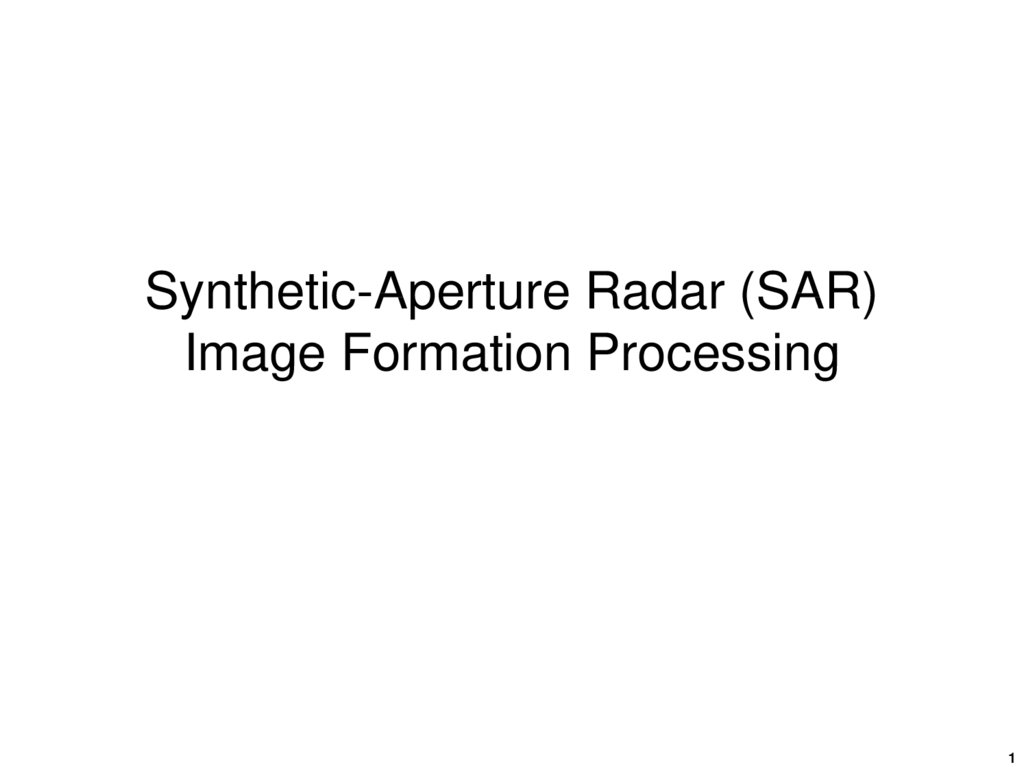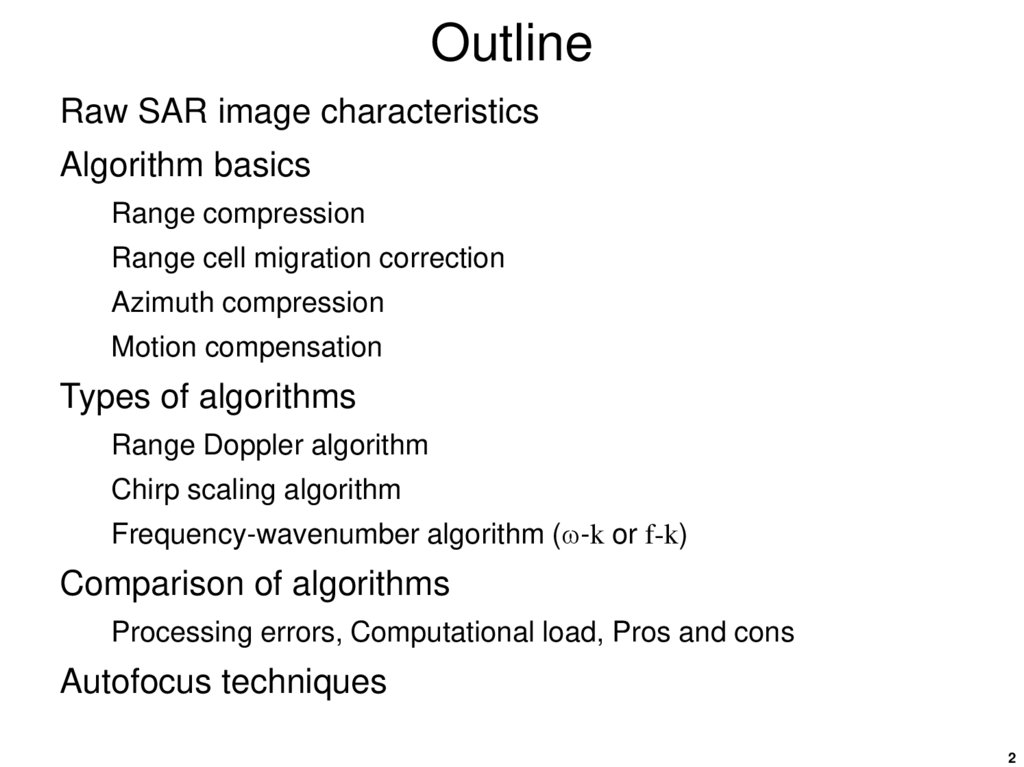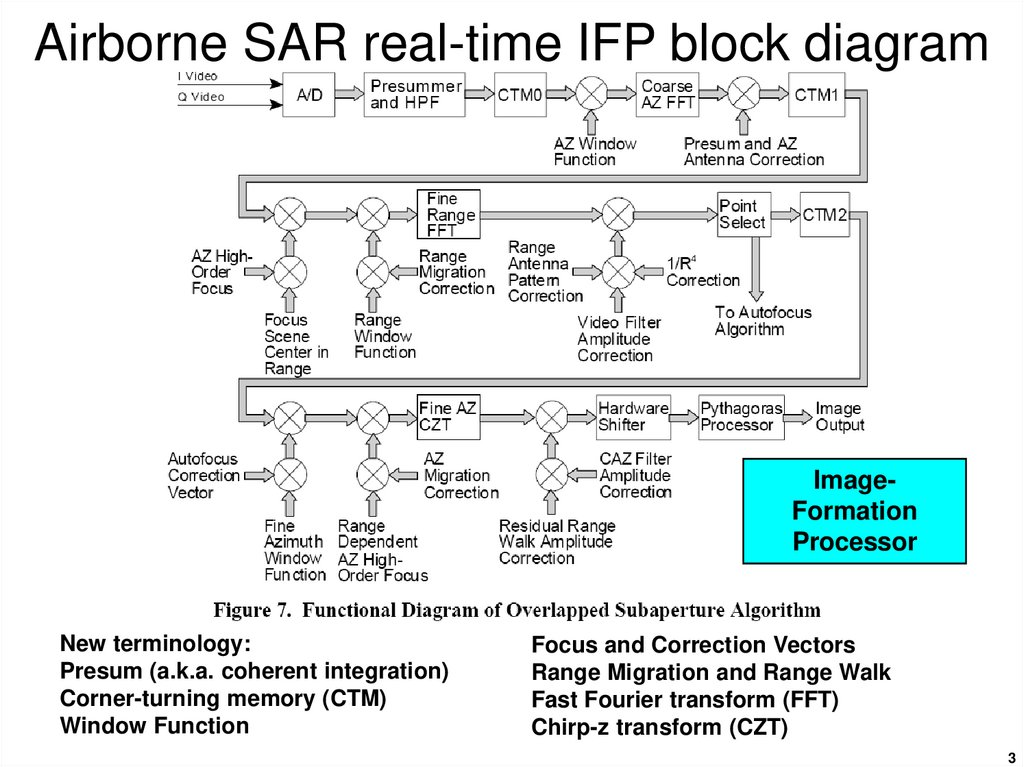# Synthetic-Aperture Radar (SAR) Image Formation Processing

1

## 2. Outline

Raw SAR image characteristics
Algorithm basics
Range compression
Range cell migration correction
Azimuth compression
Motion compensation
Types of algorithms
Range Doppler algorithm
Chirp scaling algorithm
Frequency-wavenumber algorithm ( -k or f-k)
Comparison of algorithms
Processing errors, Computational load, Pros and cons
Autofocus techniques
2

## 3. Airborne SAR real-time IFP block diagram

ImageFormation
Processor
New terminology:
Presum (a.k.a. coherent integration)
Corner-turning memory (CTM)
Window Function
Focus and Correction Vectors
Range Migration and Range Walk
Fast Fourier transform (FFT)
Chirp-z transform (CZT)
3

4

5

6

7

8

9

## 10. Demodulated baseband SAR signal [from Digital processing of synthetic aperture radar data, by Cumming and Wong, 2005]

Time domain representation
After removing the radar carrier cos(2p fot) from the
received signal, the demodulated, complex, baseband
signal from a single point target can be represented as
where
t : range (fast) time, s
: azimuth (slow) time relative to the time of closest approach, s
Ao:
wr(t ):
wa( ):
R( ):
c :
fo:
Kr :
complex constant
envelope of the transmitted radar pulse
antenna’s azimuth beam pattern
slant range in time domain, m
beam center crossing time relative to the time of closest approach, s
carrier frequency, Hz
FM rate of transmitted pulse chirp, Hz/s
10

## 11. Demodulated baseband SAR signal

includes R-4 and
target RCS factors
transmit
waveform
amplitude
antenna gain variation
over synthetic aperture
range-dependent
phase component
transmitted chirp waveform
The instantaneous slant range is
where
Vr : effective radar velocity (a positive scalar), m/s
Ro: slant range at closest approach, m
11

## 12. SAR signal spectrum [from Digital processing of synthetic aperture radar data, by Cumming and Wong, 2005]

Frequency-domain represention
For reasons of efficiency, many SAR processing algorithms
operate in the frequency domain.
For the low-squint case, the two-dimensional frequency
spectrum of the received SAR signal is
where 2df, the phase function in the two-dimensional
frequency domain, is
and Ka´, the azimuth FM rate in the frequency domain, is`
12

## 13. SAR signal spectrum

Also
ft :
Fr :
f :
f c :
range frequency, Hz, where –Fr /2 ft Fr /2
range sampling frequency, Hz
azimuth (Doppler) frequency, Hz
absolute Doppler centroid frequency, Hz
Wr(ft ) : envelope of the radar data’s range spectrum
Wa(f ) : envelope of the antenna’s beam pattern Doppler spectrum
The relationship between azimuth time to frequency is
where
13

## 14. SAR signal spectrum

envelope of the
range spectrum
antenna’s beam pattern
envelope in Doppler spectrum
phase function in twodimensional frequency domain
term due to
azimuth chirp
term due to
transmitted chirp
range-dependent
phase component
14

## 15. Matched filter processing

Given an understanding of the characteristics of the ideal
SAR signal, an ideal matched-filter can be applied using
correlation to produce a bandwidth limited impulse
response.
However this process has limitations as the characteristics
of the ideal matched-filter varies with the target’s position in
range and azimuth.
So while such correlation processing is theoretically
possible, it is not computationally efficient and is not
appropriate when large-scale image-formation processing
is required, e.g., from a spaceborne SAR system.
15

## 16. Range Doppler domain spectrum [from Digital processing of synthetic aperture radar data, by Cumming and Wong, 2005]

Range Doppler-domain representation
The range-Doppler domain is useful for range-Doppler
image formation algorithms.
The range-Doppler domain signal is
where rd, the azimuth phase function in the range-Doppler
domain, is
and Rrd(f ), the slant range in the range-Doppler domain,
represents the range cell migration in this domain
16

17

## 18. Range-dependent range migration

azimuth bandwidth
transmitted pulse bandwidth
azimuth time when target perpendicular
azimuth time when target in epicenter of azimuth
signal
tv( ) : time delay between Tx and Rx signal, = 2R( )/c
R( o ) : azimuth time-dependent distance
Baz :
Br :
o :
c :
18

19

20

21

## 22. Range-Doppler algorithm

Range cell migration
compensation (RCMC) is
performed in the range-Doppler
domain. Families of target
trajectories at the same range
are transformed into a single
trajectory that runs parallel to
the azimuth frequency axis.
RCMC: range cell migration compensation
SRC: secondary range compression
22

## 23. Range-cell migration compensation

Part of the migration
compensation requires a
re-sampling of the rangecompressed pulse using
an interpolation process.
23

## 24. Chirp scaling algorithm

The range-Doppler algorithm was the first digital algorithm
developed for civilian satellite SAR processing and is still
the most widely used.
accuracy secondary-range compression in high-squint and
wide-aperture cases) prompted the development of the
chirp-scaling algorithm to eliminate interpolation from the
range-cell migration compensation step.
As the name implies it uses a scaling principle whereby a
frequency modulation is applied to a chirp-encoded signal
to achieve a shift or scaling of the signal.
24

25

26

27

28

29

30

## 31. Omega-K algorithm (WKA)

The chirp-scaling algorithm assumes a specific form of the
SAR signal in the range Doppler domain, which involves
approximations that may become invalid for wide apertures
or high squint angles.
The Omega-K algorithm uses a special operation in the
two-dimensional frequency domain to correct range
dependent range-azimuth coupling and azimuth frequency
dependence.
The WKA uses a focusing step wherein a reference
function is multiplied to provide focusing of a selected
range. Targets at the reference range are correctly focused
while targets at other ranges are partially focused.
Stolt interpolation is used to focus the remainder of the
targets.
31

## 32. Omega-K algorithm (WKA)

Illustration of the range/azimuth cross coupling using the
raw phase history from a point target.
Range-cell migration introduces a phase change into the
azimuth samples in addition to the normal phase encoding.
The RCM cross coupling creates an additional azimuth
phase term which affects the azimuth FM rate.
From chirp pulse
compression example
a2
s( t )
cos 2 p f C T k T t 0.5 k T 2
2
Range-dependent
phase terms
32

33

34

35

36

## 37. Comparison of IFP algorithms

Hyperb: hyperbolic
P.S.: power series, i.e., parabolic
Azim MF:azimuth matched filter
RCMC: range cell migration correction
SRC: secondary range compression
37

## 38. Motion compensation

Imperfect trajectories during SAR data collection will distort
the data set resulting in degraded images unless these
imperfections are removed.
Removal of the effects of these imperfections is called
motion compensation.
Motion compensation requires precise knowledge of the
antenna’s phase center over the entire aperture.
For example vertical velocity will introduce an additional Doppler shift into
the data that, if uncompensated, will corrupt along-track processing.
Similarly a variable ground speed will result in non-periodic along-track
sampling that, if uncompensated, will also corrupt along-track processing.
Knowledge of the antenna’s attitude (roll, pitch, yaw
angles) is also important as these factors may affect the
illumination pattern as well as the position of the antenna’s
phase center.
38

## 39. Motion compensation

To provide position and attitude knowledge various
instruments are used
Gyroscopes (mechanical or ring-laser)
Accelerometers
39

40

## 41. Motion compensation

In addition to position and attitude knowledge acquired
from various external sensors and systems, the radar
signal itself can provide information useful in motion
compensation.
The Doppler spectrum can be used to detect antenna
pointing errors.
The nadir echo can be used to detect vertical velocity (at
least over level terrain).
41

## 42. Autofocus

Just as non-ideal motion corrupts the SAR’s phase history,
the received signal can also reveal the effects of these
motion imperfections and subsequently cancel them.
This process is called autofocus.
Various autofocus algorithms are available
Map drift
Phase difference
Inverse filtering
Prominent point processing
Many of these techniques exploit the availability of a highcontrast point target in the scene.
42

43

44

45

46